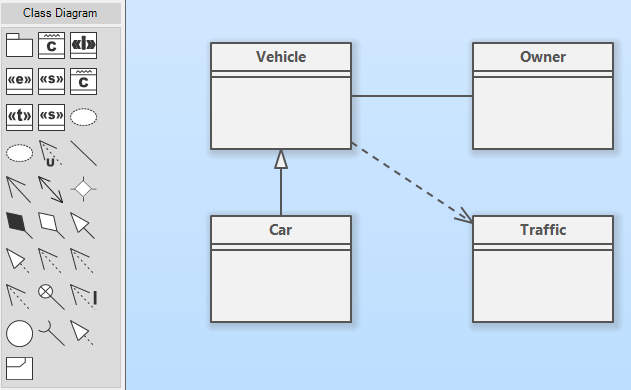# How Do I Create a UML Diagram?

UML diagrams are a standard method for describing models and processes. The UML notation provides diagrams for static structure definition as well as for behavior and process modeling. In this tutorial, you will find out how to create UML diagrams.

## How do I create a UML diagram?

UML diagrams are a standard method for describing models and processes. The UML notation provides diagrams for static structure definition as well as for behavior and process modeling. In this tutorial, you will find out how to create UML diagrams.

## Choose the Appropriate Diagram Type

First, decide whether your diagram describes a static structure or some kind of behavior or process. If you want to design a static model, choose one of the structural UML diagram types:

To describe a process, behavior, or system dynamics create one of these diagrams:

## Create New Diagram

Before you start the actual creative process, you need to add an empty UML diagram to your project. Click on the Plus button in the tab bar or click on the button Add New Diagram on the Project tab (Content group). Choose the desired diagram type from the UML group. A new diagram will be created and added to the project. The diagram editor with the diagram will open in a new tab.

Now you can choose the tools from the toolbox (by default on the left side of the diagram editor). The UML diagram elements are available in the toolbox group named by the chosen diagram types (e.g. if you are editing a UML class diagram, the toolbox with element tools is named Class Diagram).UML class diagram editor

## Draw UML Diagram

Place the diagram elements into your diagrams using drag&drop - drag a desired element type button from the toolbox to the diagram editor canvas and drop it over the desired position. Start from the core idea and add more and more details. Define relationships between the elements. If something is unclear from the actual notation, use the comment element to add an explanation to your diagram. This way you can build the whole diagram.

If the diagram is too large that it is hard to read it without scrolling, consider splitting the diagram into multiple smaller diagrams. You can add a nested diagram to a higher-level element.

Comment